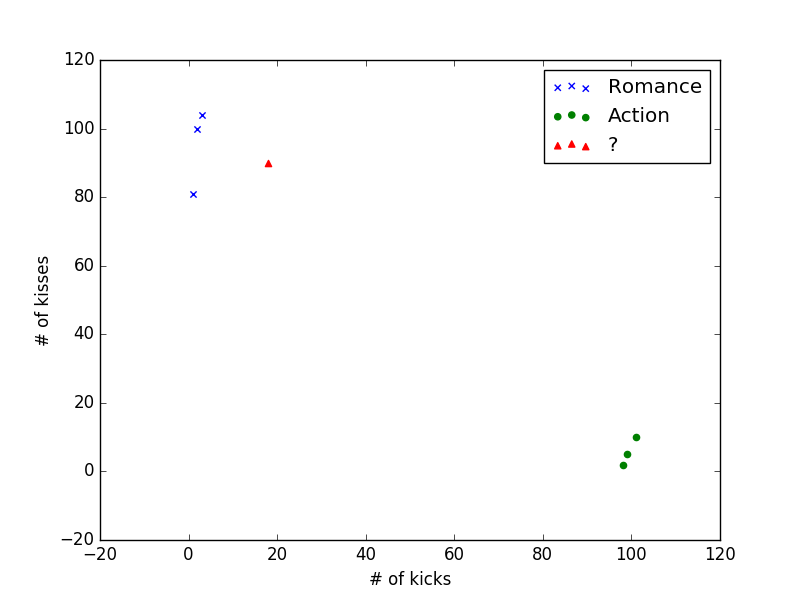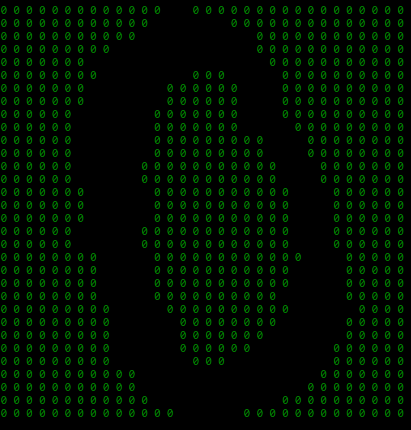# 机器学习实战笔记(1)——k-近邻算法

## 3. 爱情片还是动作片

California Man 3 104 爱情
He’s Not Really into Dudes 2 100 爱情
Beautiful Woman 1 81 爱情
Robo Slayer 3000 99 5 动作
Amped II 98 2 动作
X 18 90 ?$d = \sqrt{(x - x_0)^2 + (y - y_0)^2}$

California Man 20.5
He’s Not Really into Dudes 18.7
Beautiful Woman 19.2
Robo Slayer 3000 117.4
Amped II 118.9

from numpy import *
import operator

def classify(input, train_set, labels, k):
data_size = train_set.shape
diff_mat = tile(input, (data_size, 1)) - train_set
square_diff_mat = diff_mat ** 2
square_distances = square_diff_mat.sum(axis=1)
distances = square_distances ** 0.5
sorted_distances = distances.argsort()
class_count = {}
for i in range(k):
label = labels[sorted_distances[i]]
class_count[label] = class_count.get(label, 0) + 1
sorted_class_count = sorted(class_count.iteritems(), key=operator.itemgetter(1), reverse=True)
return sorted_class_count


pip install numpy


def test():
data_set = array([[3, 104], [2, 100], [1, 81], [101, 10], [99, 5], [98, 2]])
labels = ['Romance', 'Romance', 'Romance', 'Action', 'Action', 'Action']

print classify([18, 90], data_set, labels, 3)


## 4. 约不约

1. 每年获得的飞行常客的里程数
2. 玩游戏所消耗的时间百分比
3. 每周消费的冰淇淋公升数

40920	8.326976	0.953952	3
14488	7.153469	1.673904	2
26052	1.441871	0.805124	1
...


def read(filename):
fr = open(filename)
data_set = zeros((line, 3))
labels = []
fr = open(filename)
index = 0
line_list = line.strip().split('\t')
data_set[index, :] = line_list[0:3]
labels.append(int(line_list[-1]))
index += 1;
return data_set, labels


$new = \frac{old - min}{max - min}$

format函数来完成这件事：

def format(data_set):
min = data_set.min(0)
max = data_set.max(0)
step = max - min
new_data_set = zeros(shape(data_set))
line = data_set.shape
new_data_set = data_set - tile(min, (line, 1))
new_data_set /= tile(step, (line, 1))
return new_data_set


def test():
data_set = format(data_set)
data_count = data_set.shape
train_set = data_set[test_count:, :]
label_set = labels[test_count:]
error_count = 0
for i in range(test_count):
test_list = data_set[i, :]
label = classify(test_list, train_set, label_set, 3)
if not label == labels[i]:
error_count += 1
return error_count / float(test_count)


def judge(x1, x2, x3):
min = data_set.min(0)
max = data_set.max(0)
input = (array([x1, x2, x3]) - min) / (max - min)
return classify(input, format(data_set), labels, 3)


## 5. 手写识别

00000000000001100000000000000000
00000000000011111100000000000000
00000000000111111111000000000000
00000000011111111111000000000000
00000001111111111111100000000000
00000000111111100011110000000000
00000001111110000001110000000000
00000001111110000001110000000000
00000011111100000001110000000000
00000011111100000001111000000000
00000011111100000000011100000000
00000011111100000000011100000000
00000011111000000000001110000000
00000011111000000000001110000000
00000001111100000000000111000000
00000001111100000000000111000000
00000001111100000000000111000000
00000011111000000000000111000000
00000011111000000000000111000000
00000000111100000000000011100000
00000000111100000000000111100000
00000000111100000000000111100000
00000000111100000000001111100000
00000000011110000000000111110000
00000000011111000000001111100000
00000000011111000000011111100000
00000000011111000000111111000000
00000000011111100011111111000000
00000000000111111111111110000000
00000000000111111111111100000000
00000000000011111111110000000000
00000000000000111110000000000000def read(train_dir):
pic_list = os.listdir(train_dir)
train_set = zeros((len(pic_list), 1024))
labels = []
for index, img in enumerate(pic_list):
labels.append(int(img))
return train_set, labels


read函数有调用了read_img函数，它用来读取一张图片，并把该图片转换为一个1x1024的向量。

def test(test_dir):
pic_list = os.listdir(test_dir)
error_count = 0
for index, img in enumerate(pic_list):